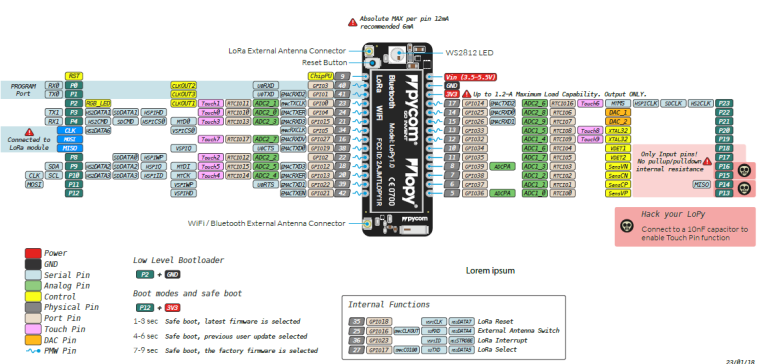# How to correctely use Analog input

• Helloo
I am new with Pycom and I need to use an analog pin to get the value of 3 identical moisture sensors and one capacitive sensor.

I would like to measure a range of 0-1023, so to make sure to have this range, I should select bit=10.

I do not want to be dependent of the pin outpt voltage then I think, it would be good to use a voltage reference with

``````adc.vref(*, vref)
``````

I am not sure about several thinks. First, I found this exemple:

adc = ADC(0)

but I also found this

``````adc = ADC(0)
``````
``````adc = ADC(bits=10)
``````
``````adc = ADC()
``````
``````adc.init(bits=10)
``````

then I am confused how to correctly froce the bit range from 0-1023

I suppose all does the same if bits=10 is the default. I would prefere use

``````adc=ADC(bits=10)
``````

but is it really correct, or should I consider

``````adc = ADC()
adc.init(bits=10
``````

Secondely
I would like to set an external voltage reference.
Here, calibration it state, we need to configure by measuring P22. But P22 is SDA pin as well and I need to use I2C.

So if I do that (considering, I measure 1.1V at P21)

``````adc = ADC()
adc.init(bits=10)
adc.vref_to_pin('P22')
adc.vref(1100)
``````

The above code will not disturb the SDA pin, when I will connect an I2C sensor?
Does my above code is correct, regarding vref?

With Arduino , there is 3V pin, which is always at 3.3V. I can solder a wire from the 3V pin to the aref pin to have 3.3V as a reference.
With LoPy4, is there a such pin, that we can trust the output voltage and use it a vref?

Lastely
I do not understand what does exately attenuation level

``````# ADC.ATTN_11DB
wm_1 = adc.channel(pin='P13', attn = machine.ADC.ATTN_11DB)
``````

IS depend of the output voltage? I heard ATTN_11DB is from 0-3V and it look like what I need...

Thank for your clarification
Cheers
Pierre

• @pierrot10 Vref is an internal voltage independent from the supply voltage of the extension board. And it is, like @seb said, just used for correcting the values returned by the API. The default assumption about VRef is 1100 mV. You may also completely ignore it, and just use wm_1.value() to get the raw value; especially since your application does not seem to be a priori linked to any standard physical quantity. You have have to make your interpretation table of the returned values yourself, and it does not matter if that is mV -> humidity or number -> humidity.

• @seb
Dear Seb, thank for your reply and sorry to reply so late. I was busy with web project. Now I resumed my lovely pycom board and continue about moisture sensore on analog pin.

For the fist question. It's cear for me. I have a doubt about the second.

``````you can output Vref onto P6 P21 or P22, take a measurement with a multimeter, then record the value in mV
``````

So if I understand the doc, I should add the following code

``````#Run it only once, then comment the code " for ever"
if(isADCCalibrating == True):
# 1) Output Vref of P22
adc_cal = adc.vref_to_pin('P22')
print("P22 (mV):",adc_cal) # this will really print a value?
# 2) Now measure the value of P22 with a multimeter
# 3) replace the value of 1100 with the measured value
adc.vref(1100)

# 4) Now connect your sensor on pin 13 and print the value returned by your sensor
wm_1 = adc.channel(pin='P13', attn = machine.ADC.ATTN_11DB)
print("Sensor 1 (mV): ", wm_1);
``````

When is done, I can change the value isADCCalibrating to False
and I can take the value of Pin3 either as the following

``````print("Sensor 1",wm_1())
``````

or should I always call

``````wm_1 = adc.channel(pin='P13', attn = machine.ADC.ATTN_11DB)
print("Sensor 1 (mV): ", wm_1);
``````

(I have a doubt, In the code I wrote some month ago, I found wm1(), but it can be an error :) )

For my last question, I again have a doubt.

My extantion board is powered with a 3.7V, so the reference will be over 1100, that correct? (I have not measure it yet. Sorry). In any case and inevitabely, I have to use the following (11db)

``````machine.ADC.ATTN_11DB
``````

Sepcialy if the measured value (with the voltmeter) is above 1100.

I supposed depending og the measured value, I will have to choose
attn=ADC.ATTN_6DB
attn=ADC.ATTN_11DB
etc..

For sure, when I will measure P22, it will clear, but I do not have the material right now.

Thank a lot for your clarification!
Pierrot

(I will try all as soon I will get back my multimter...)

• @jclo13
I2C can be initialized on differnet pins but as you pointed default are P9 (SDA) and P10 (SCL).

• @pierrot10 I am a little confused, I suppose that you are using a lopy, right?, well according to the lopy pinout, the P22 is not an SDA pin, the SDA pin is P9, or maybe I am making a mistake.• Hi,

To answer your first question, the following two blocks of code are functionally, identical.

``````adc = ADC(bits=10)
``````
``````adc = ADC()
adc.init(bits=10)
``````

If you require 10-bit resolution then please specify this rather than relying on a default behaviour. This way if anything changes in the future your code will still work as expected.

Second, with regards to the ADC calibration, this is something you only need to do once, you can output Vref onto `P6` `P21` or `P22`, take a measurement with a multimeter, then record the value in mV. After this you never need to output the reference voltage unless you want to use it for something.

Finally, about antennuation. the ADC on the ESP32 ranges from 0 to 1v, therefore attenuation of the input signal is required to read voltages above this. The following levels are supported
0dB = 0-1V
2.5dB = 0-1.33V
6db = 0-2v
11db = 0 - 3.55V